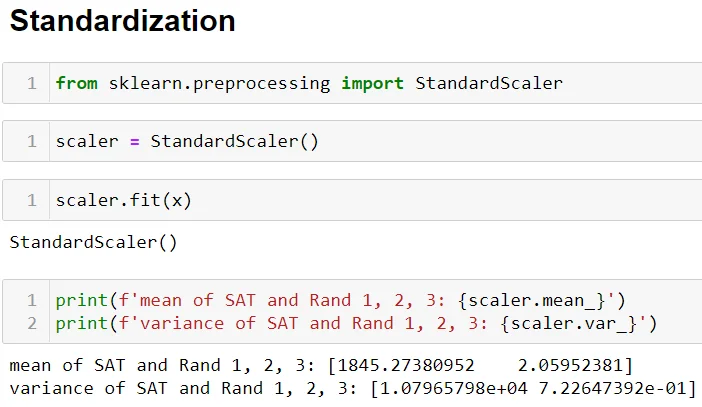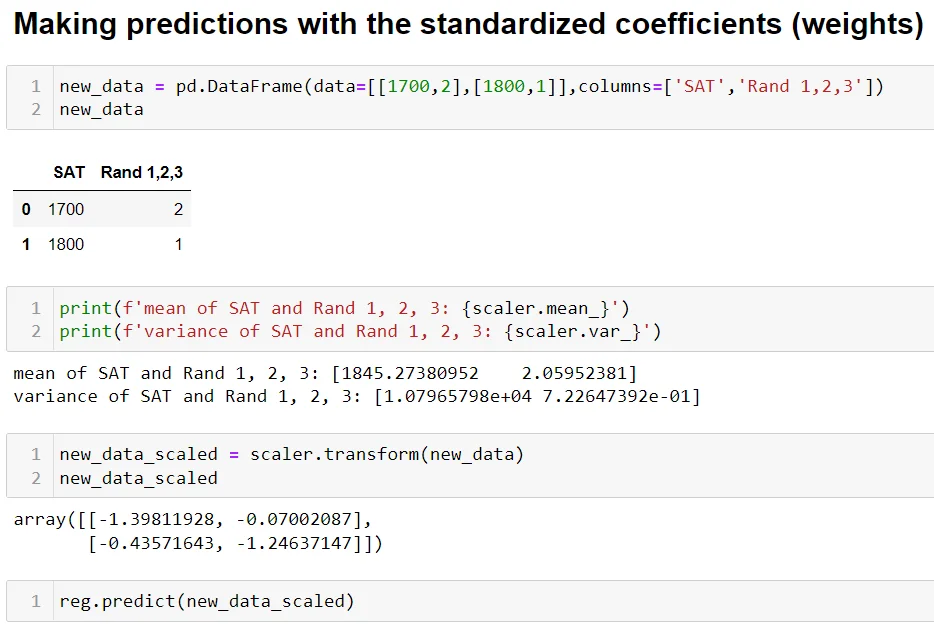31 Aug 2022

Posted on:

31 Aug 2022

0

# Scaling New Data using Scaler.Transform

new_data_scaled = scaler.transform(new_data)
which mean and std deviation will be used to transform the new_data?
- Mean and Std Deviation from scaler.fit(x) at the beginning of this exercise?

Instructor
Posted on:

31 Aug 2022

0

Hey,

Thank you for reaching out!

That is exactly right. At the beginning of the notebook, we use our "training" data, `data[['SAT','Rand 1,2,3']]`, to calculate the mean and the standard deviation of each of the features. The result is as follows (the standard deviation is the square root of the variance):Towards the end of the notebook, when making predictions, we use the same `scaler` object (storing the same mean and variance values) to predict the outcome:In fact, it is very important to use the `StandardScaler()` object storing the mean and variance of the training data. The mean and variance of the test data, which in our case is `[1700, 2]` and `[1800, 1]`, should remain unknown to the `scaler` object. That is to say, we use the mean and variance of the training data to transform the test data.

Hope this helps!

Kind regards,
365 Hristina